# HC Verma Solutions Class 12 Chapter 12 Magnetic Field

HC Verma Solutions Class 12 Chapter 12 Magnetic Field will help students understand the chapter in a much better way. The solutions will further enable them to study at their own pace in a much more streamlined manner. The solutions come answers to all the exercises as well as solved examples that will make studying this unit easy. Students can also increase their question-solving efficiency by referring to the solutions.

One major benefit of HC Verma Solutions is that complex questions on Magnetic Field can be easily solved using it. These solutions will also help students understand the concepts in depth and develop a strong base. In addition, students of 12th grade can use this study material for various competitive exams like IIT JEE and NEET.

## Key Magnetic Field Topics

Students learning about the magnetic field will come across important topics like;

• Definition Of Magnetic Field
• Relation Between Electric and Magnetic Fields
• Motion Of A charged Particle In A Uniform Magnetic Field
• Magnetic Force On a Current-carrying Wire
• Torque On A Current Loop

## Class 12 Important Questions In Chapter 12

1. Is it possible to accelerate a charged particle by a magnetic field? Will the speed be increased or does it remain uniform?

2. Why does a magnetic field exert a force on a current-carrying wire when its net charge is zero?

3. The velocity of a charged particle moving in a uniform magnetic field at some instant makes an acute angle with the magnetic field. During this, what will happen to the path of the particle?

(a) moves in a straight line (b) in a circle (c) in a helix with uniform pitch (d) in a helix with nonuniform pitch.

4. You want to apply a magnetic field to an electron moving along the positive X-axis so that the electron may reverse its direction and move parallel to the negative X-axis. Where should the magnetic field be applied along?

(a) Z-axis (b) Y-axis (c) Z-axis only (d) Y-axis only

5. Do you think that a current loop can stay without rotating in a uniform magnetic field? Describe the orientation of the loop?

## HC Verma Solutions Vol 2 Magnetic Field Chapter 12

Question 1: An alpha particle is projected vertically upward with a speed of 3.0 × 104 km s–1 in a region where a magnetic field of magnitude 1.0 T exists in the direction south to north. Find the magnetic force that acts on the α-particle.

Solution:

Magnetic field, B = 1.0 T

Upward speed of the alpha particle = v = 3 × 104 km s-1

Charge on the alpha particle = q = 2e

where, e = charge of an electron

=> q = 2 × 1.6 × 10−19 C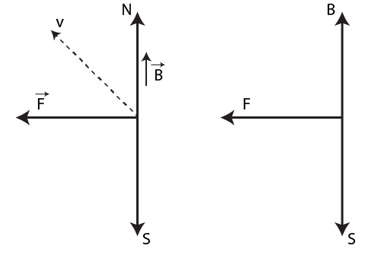Magnetic force acting on the alpha particle:

F = qv x B sin 90o

= 2 x 1.6 x 10-19 x 3 x 107 x 1

= 9.6 × 10−12 N towards west.

Question 2: An electron is projected horizontally with a kinetic energy of 10 keV. A magnetic field of strength 1.0 × 10–7 T exists in the vertically upward direction.

(a) Will the electron deflect toward right or towards left of its motion?

(b) Calculate the sideways deflection of the electron in travelling through 1m. Make appropriate approximations.

Solution:

K.E. of the electron when projected towards horizontal direction = 10 keV = 1.6 × 10−15 J

Magnetic field = B = 1 × 10−7 T

(a) The direction of electron deflection can be found by the right-hand screw rule. Since magnetic field is vertically upward, So, electron will be deflected towards left.

(b) We know, K.E. = (1/2) mv2

or v = √[2(K.E.)/m]

Again, we know relation, F = qvB sinθ

Also, by F = ma [using Newton’s second law of motion]

Using above relations, we have

a = [qv x B]/m

Applying equation of motion:

s = ut + (1/2) at2

Since, the initial velocity is zero, so = u = 0

using value of “a”

=> s = (1/2) [[qv x B]/m] t2

Also, we know time (t) = distance/velocity

or t = x/v

=> s = (1/2) [[qv x B]/m] (x/v) 2

On substituting the value of v, we get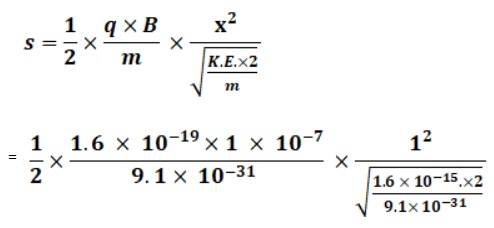We know,

Mass of an electron = 9.1× 10-31 kg

Charge on an electron = 1.60 × 10-19

=> s = 1.5 x 10-2 cm

Question 3: A magnetic field of (4.0 x 10-3 $\vec k$)T exerts a force of (4.0 $\vec i$+ 3.0$\vec j$) x 10-10N on a particle having a charge of 1.0 x 10-9 C and going in the X-Y plane. Find the velocity of the particle.

Solution:

We are given with force and magnetic field.

Electric charge on the particle = q = 1 × 10−9 C (going in the X-Y plane)

x-component of force= Fx = 4 × 10−10 N and

y-component of force = Fy = 3 × 10−10 N

F = qvB sin θ

or v = F/[q B sinθ]

Motion along x-axis:

vx = [4×10-10]/[1×10-9x4x10-3]

= 100 ms-1

Similarly, vy = [3×10-10]/[1×10-9x4x10-3] = 75 ms-1

Therefore, velocity of the particle = -75$\vec i$ + 100 $\vec j$ ms-1

Question 4: An experimenter’s diary reads as follow: “a charged particle is projected in a magnetic field of (7.0 $\vec i$- 3.0 $\vec j$) x 10-3 T. The acceleration of the particle is found to be (x $\vec i$+ 7.0 $\vec j$) x 10-6 m/s2. The number to the left of “i” in the last expression was not readable. What can this number be?

Solution:

Magnetic field = B = (7.0 $\vec i$ – 3.0 $\vec j$) x 10-3 T.

Acceleration of the particle = a = (x $\vec i$+ 7.0$\vec j$ ) x 10-6 m/s2

Magnetic force always acts perpendicular to the motion of the particle, this implies B and a are perpendicular to each other.

So, $\vec B . \vec a$= 0

=> (7x) x 10-3 x 10-6 – 3 x 7 x 10-3 x 10-6 = 0

=> x = 3

So, Acceleration of the particle = a = (3 $\vec i$+ 7.0$\vec j$ ) x 10-6 m/s2

Question 5: A 10 g bullet having a charge of 4.00 μC is fired at a speed of 270 ms–1 in a horizontal direction. A vertical magnetic field of 500 μT exists in the space. Find the deflection of the bullet due to the magnetic field as it travels through 100 m. Make appropriate approximations.

Solution:

Speed of the bullet in horizontal direction = v = 270 m/s

Vertical magnetic field = B = 500 μT = 500× 10-6 T

m = 10g = 10-3 Kg and q = 4.00 μC =10-6 C

Since distance travelled by the bullet = d = 100 m

=> time = distance/velocity = 100/270 s …(1)

We know, F = qvB sinθ and F = ma

=> qvB sinθ = ma

=> a = qvB/m ….(2)

Deflection due to magnetic field in time t,

y = (1/2)at2

using (1) and (2), and substitute all the given values

= (1/2) x [4×10-6 x270x500x10-6]/[10×10-3] x (100/270)2

= 3.7 x 10-6 m

Question 6: When a proton is released from rest in a room, it starts with an initial acceleration a0 towards west. When it is projected towards north with a sped v0, it moves with an initial acceleration 3a0 towards west. Find the electric field and the minimum possible magnetic field in the room.

Solution:

When a proton is released from rest in a room, it starts with an initial acceleration a0 towards west. (Given)

Using coulomb’s force F and Newton’s second law of motion

F = qE and F = ma0

Equating both the equations, we have

ma0 = qE

=> E = ma0/q [acts towards west]

Again, we know, F = qvB sinθ

=> B = F/qv0

An electrical force acts on the proton in the west direction, producing an acceleration a0 on the proton.

Initially, the proton was moving at velocity v, so “B” also acted on the proton.

So, the magnetic force is the only reason for the change in the acceleration of the proton.

Change in acceleration towards west due to the magnetic force acting on it:

∆a = 3a0 − a0 = 2a0

So, F = m(2a0)

Hence, B = (2ma0)/qv0

Question 7: Consider a 10-cm long portion of a straight wire carrying a current of 10 A placed in a magnetic field of 0.1 T making an angle of 53° with the wire. What magnetic force does the wire experience?

Solution:

Magnetic Force on a Current carrying wire = F = BiL sinθ

Where B= magnetic field, I = current and L = length of the wire

at θ = 53o, sinθ = sin 53o = 0.798

F = 10 x 10x 10-2 x 0.1 x 0.798 = 0.08 (approx)

The direction of force can be found using Fleming’s left-hand rule.

When a current carrying conductor is placed in a magnetic field, a force acts on the conductor in a direction perpendicular to the directions of the current and the magnetic field.

Direction of the magnetic force is perpendicular to both the wire and the magnetic field.

Question 8: A current of 2A enters at the corner d of a square frame abcd of side 20 cm and leaves at the opposite corner b. A magnetic field B = 0.1 T exists in the space in a direction perpendicular to the plane of the frame as shown in figure. Find the magnitude and direction of the magnetic force on the four sides of the frame.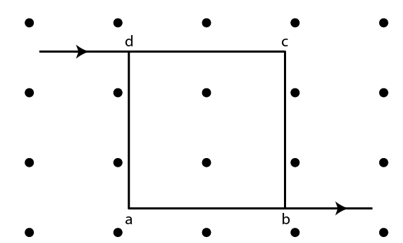Solution:

Magnetic Force on a Current carrying wire = F = BiL sinθ …(1)

Angle between the frame and magnetic field, θ = 90°

substitute given values in (1), we have

F = 22 x 20 x 10-2 x 0.1

= 0.02 N

The direction of force can be found using Fleming’s left-hand rule.

When a current carrying conductor is placed in a magnetic field, a force acts on the conductor in a direction perpendicular to the directions of the current and the magnetic field.

So, direction of magnetic force will be towards the left.

For wires along sides, dc and ab,

F = 22 x 20 x 10-2 x 0.1 = 0.02 N

The direction of force can be found using Fleming’s left-hand rule.

The direction of magnetic force will be downwards.

Question 9: A magnetic field of strength 1.0 T is produced by a strong electromagnet in a cylindrical region of radius 4.0 cm as shown in figure. A wire, carrying a current of 2.0 A, is placed perpendicular to and intersecting the axis of the cylindrical region. Find the magnitude of the force acting on the wire.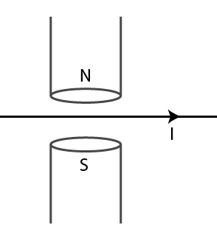Solution:

Magnetic Force on a Current carrying wire = F = BiL sinθ …(1)

Angle between the wire and magnetic field, θ = 90° and length, l = 2r = 8 x 10-2 m

substitute given values in (1), we have

F = 2 x 8 x 10-2 x 1.0T x 1

= 0.16 N

Question 10: A wire of length ℓ carries a current i along the x-axis. A magnetic field exits which is given as B = B0 ($\vec i+ \vec j + \vec k$)T. Find the magnitude of the magnetic force acting on the wire.

Solution:

Given : current is passing along the X-axis.

Magnetic field in vector form = B = Bo( $\vec i+ \vec j + \vec k$)T

Electric current through the wire = i $\vec i$A

Magnetic Force on a Current carrying wire = F = BiL sinθ

= Bo ( $\vec i+ \vec j + \vec k$)T x i$\vec i$ x l sinθ

Since current, I, is along x- axis, $\vec i \times \vec i$ = 0

F = Bo ( $\vec i+ \vec j + \vec k$)T x i$\vec i$ x l

= Bo il k – Bil j

Magnitude of the magnetic force = |F| = √ [(Bo il )2 + (Bo il)2]

= √2 Bo il

Question 11: A current of 5.0 A exists in the circuit shown in figure. The wire PQ has a length of 50 cm and the magnetic field in which it is immersed has a magnitude of 0.20 T. Find the magnetic force acting on the wire PQ.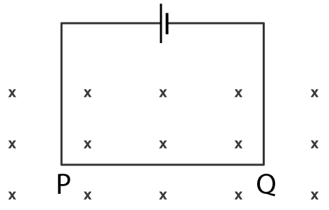Solution:

The direction of magnetic field is perpendicular to the plane and it is going into the plane.

Angle between the plane of the wire and the magnetic field = θ = 900

we know, F = BiL sinθ

F = 5 x 50 x 10-2 x 0.2 x 1

= 0.50 N

The direction of the force can be determined using Fleming’s left rule.

When a current-carrying conductor is placed in a magnetic field, a force acts on the conductor in a direction perpendicular to the directions of the current and the magnetic field. Thus, the direction of the magnetic force in the paper plane is directed upwards.

Question 12: A circular loop of radius a, carrying a current i, is placed in a two-dimensional magnetic field. The centre of the loop coincides with the centre of the field figure. The strength of the magnetic field at the periphery of the loop is B. Find the magnetic force on the wire.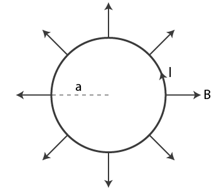Solution:

Let “a” be the radius of circular loop.

Circumference = Length of the loop = 2πa

As per question, loop is placed in a two-dimensional magnetic field. And the centre of the loop coincides with the centre of the field.

Direction of magnetic is radially outwards.

[strength of the magnetic field at the periphery of the loop is B]

The angle between the length of the loop and the magnetic field, θ = 900

we know, F = BiL sinθ

F = Bi(2πa)

The direction of the force can be determined using Fleming’s left rule.

When a current-carrying conductor is placed in a magnetic field, a force acts on the conductor in a direction perpendicular to the directions of the current and the magnetic field.

Direction of magnetic force lies perpendicular to the plane pointing inwards.

Question 13: A hypothetical magnetic field existing in a region is given by B = B0 er, where er denotes the unit vector along the radial direction. A circular loop of radius a, carrying a current i, is placed with its plane parallel to the x-y plane and the centre at (0, 0, d). Find the magnitude of the magnetic force acting on the loop.

Solution:

Let “a” be the radius of circular loop.

Length of the loop = 2πa

We are given that, the loop is placed with its plane parallel to the X−Y plane and its centre lies at (0,0, d).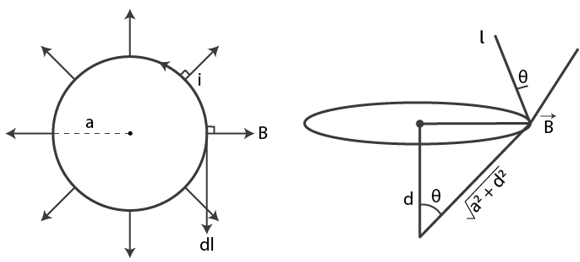Let “θ” be the angle between the length of the loop l and the magnetic field B. Then F (magnetic force) is as:

|F| = i l x B

= i(2πa x B) sinθ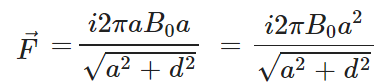Question 14: A rectangular wire-loop of width a is suspended from the insulated pan of a spring balance as shown in figure. A current i exists in the anticlockwise direction in the loop. A magnetic field B exists in the lower region. Find the change in the tension of the spring if the current in the loop is reversed.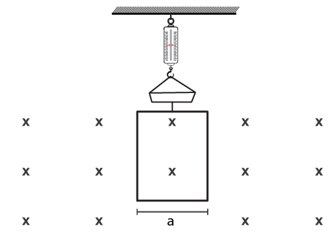Solution: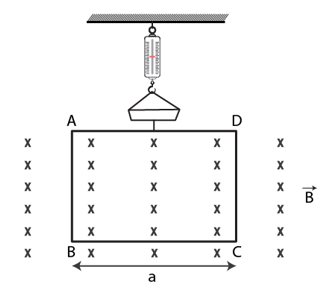From figure, Direction of the magnetic field is into the plane of the loop.

The angle between the length of the loop and the magnetic field, θ = 900

So, magnetic force = $\vec F = i\vec a \times \vec B$ = ia B sinθ

F = Bi(2πa)

Direction of force can be found using Fleming’s left-hand rule. The direction of the magnetic force will be upward. Similarly, changing the direction of current to clockwise, the force along BC, F = ia x B

Change in force = change in tension = iaB – (-iaB) = 2iaB

Question 15: A current loop of arbitrary shape lies in a uniform magnetic field B. Show that the net magnetic force acting on the loop is zero.

Solution:

Consider a square magnetic loop as below: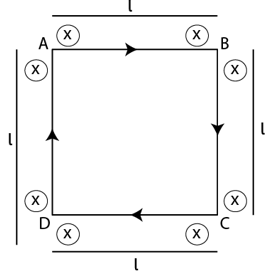Let i be the current flowing clockwise through the loop.

Direction of the magnetic field is going towards the plane of the loop.

Magnetic force = $\vec F = i\vec l \times \vec B$

F = il B Sinθ

Here θ = 90o

Let us find the direction of force using Fleming’s left-hand rule.

Force F1 acting on side AB = ilB (directed upwards)

Force F2 acting on side DC = ilB (directed downwards)

F1 and F2 are equal and in opposite direction, Both will cancel each other.

Similarly,

Force F3 acting on AD = ilB (Directed outwards, pointing towards left from AB)

Force F4 acting on BC = ilB (directed outwards, pointing towards right from BC)

F3 and F4 also cancel each other

Net force acting on the arbitrary loop is zero.

Question 16: Prove that the force acting on a current carrying wire, joining two fixed points a and b in a uniform magnetic field, is independent of the shape of the wire.

Solution:

Consider two wires of length l, one straight and the other circular.

Length of circular wire having radius “a” = 2πa

and Magnetic force = F = ilB sin(90o) = ilB

If magnetic field is pointing along the z-direction and both the wires are lying in the xy plane, the angle between the magnetic field and area vector is 90°.

For the straight wire of length l in a uniform magnetic field of strength B:

Magnetic force = F = ilB sin(90 o) = ilB

Forces are equal in both the cases. Which shows that magnetic force is independent of the shape of the wire and depends on the length and orientation of the wire.

Therefore, magnetic force is independent of the shape of the wire.

Question 17: A semicircular wire of radius 5.0 cm carries a current of 5.0 A. A magnetic field B of magnitude 0.50 T exists along the perpendicular to the plane of the wire. Find the magnitude of the magnetic force acting on the wire.

Solution:

Length of the wire = 2r = 10 cm

Direction of magnetic field is perpendicular to the plane of the wire.

=> angle between length of the wire and magnetic field, θ = 90 o

now, F = ilB

= i(2r) B

= 5 x 2 x 0.05 x 0.5

= 0.25 N

Question 18: A wire, carrying a current i, is kept in the x-y plane along the curve y = A sin(2π/λ)x . A magnetic field B exists in the z-direction. Find the magnitude of the magnetic force on the portion of the wire between x = 0 and x = λ.

Solution:

Find the magnetic force on the portion of the wire between x = 0 and x = λ.

We know ,magnetic force acting on a current carrying wire in an uniform magnetic field, F = Bil

For a differential length dl

F = Bi dl sinθ

The effective force on the whole wire due to force acting on the wire.

Integrate above equation between range 0 to λ.

=> F = i λ B.

Question 19: A rigid wire consists of a semicircular portion of radius R and two straight sections figure. The wire is partially immersed in a perpendicular magnetic field B as shown in the figure. Find the magnetic force on the wire if it carries a current i.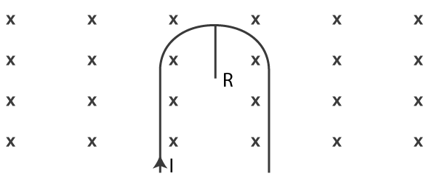Solution:

As wire is partially immersed in a perpendicular magnetic field.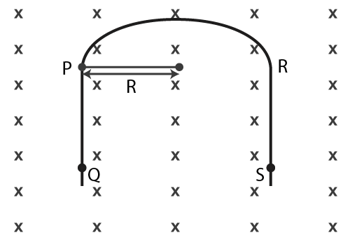From figure, PQ and RS are straight wires with a length of 1 each and the strength of the magnetic field is also the same on both wires.

direction of force) left hand can be found out.

Force acting on these wires is the same, but in opposite directions

The magnetic force on the wire PQ and the force on the wire RS are therefore the same and opposite. Both forces will cancel each other.

Therefore, only the semicircular loop PR experiences a net magnetic force.

Magnetic force in the loop PR,

F = il x B

Here l = 2r

F = 2Ri B

[As angle between the length of the wire and magnetic field, θ = 90 o]

Question 20: A straight horizontal wire of mass 10 mg and length 1.0 m carries a current of 2.0 A. What minimum magnetic field B should be applied in the region so that the magnetic force on the wire may balance its weight?

Solution:

We know, weight of an object = W = mg

Also, magnetic force acting on a current carrying wire in and uniform magnetic field,

F = Bil sinθ

We are given that, the weight of the wire should be balanced by the magnetic force acting on the wire.

=> mg = Bil sinθ

=> B = mg/il

= 10-5 x 9.82 x 1

= 4.9 x 10-5 T

Question 21: Figure shows a rod PQ of length 20.0 cm and mass 200 g suspended through a fixed point O by two threads of lengths 20.0 cm each. A magnetic field of strength 0.500 T exists in the vicinity of the wire PQ as shown in the figure. The wires connecting PQ with the battery are loose and exert no force on PQ.

(a) Find the tension in the threads when the switch S is open.

(b) A current of 2.0 A is established when the switch S is closed. Find the tension in the threads now.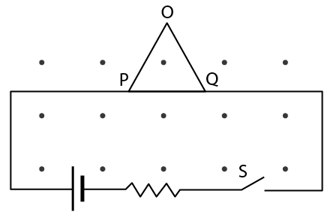Solution: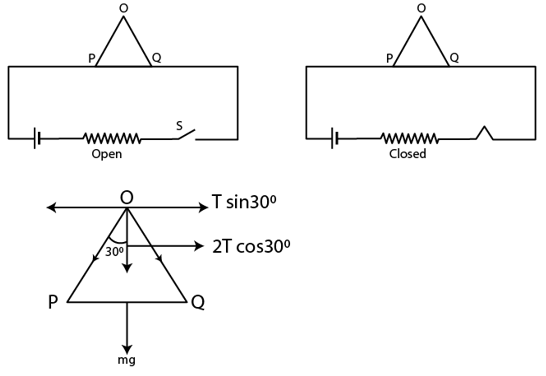(a) When the switch is open:

The weight of the rod is balanced by the tension in the rod.

=> 2T cos 300 = mg

T = mg/(2cos300)

= [0.2×9.8]/2(√3/2)

= 1.13 N

(b) When the switch is open and current flowing through the circuit = 2 A

=> 2T cos 300 = mg + iBl

T = 0.2 x 9.8 + 2 x 0.2 x 0.5

= 1.25 N

Question 22: Two metal strips, each of length ℓ, are clamped parallel to each other on a horizontal floor with a separation b between them, A wire of mass m lies on them perpendicularly as shown in figure. A vertically upward magnetic field of strength B exists in the space. The metal strips are smooth but the coefficient of friction between the wire and the floor is μ. A current i is established when the switch S is closed at the instant t = 0. Discuss the motion of the wire after the switch is closed. How far away from the strips will the wire reach?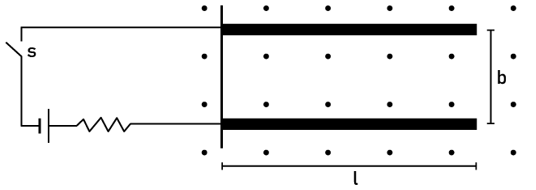Solution:

Let the wire move a distance x.

The existing magnetic field acts on the wire towards the right.

Since the coefficient of friction is zero, the distance between the wire and the strip is smooth.

Due to the influence of the magnetic force, the wire initially travels a distance that corresponds to the length of the strips. Then it travels a distance x and then a frictional force acts on the wire against its direction of movement.

So work done by the magnetic force and the frictional force will be equal.

Ff = μW

And Magnetic force due to presence of current = F = Bil

where, B= magnetic field, I = current and l = length of the wire

=> μmg x = Bib x l

=> x = iblB/μmg

Question 23: A metal wire PQ of mass 10 g lies at rest on two horizontal metal rails separated by 4.90 cm figure. A vertically downward magnetic field of magnitude 0.800 T exists in the space. The resistance of the circuit is slowly decreased and it is found that when the resistance goes below 20.0 Ω, the wire PQ starts sliding on the rails. Find the coefficient of friction.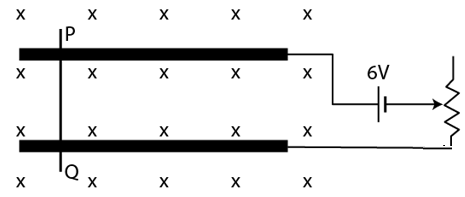Solution:

When the resistance of the circuit is slowly decreased below 20.0Ω the wire end PQ starts sliding on the metallic rails.

Current i from ohm’s law becomes, i = v/R = 6/20 A

From Fleming’s left-hand rule, magnetic force will act towards the right.

This magnetic force causes the wire to slide on the rails.

The frictional force present on the surface of the metal rails tries to counteract this motion of the wire.

When the wire begins to slide on the rails, the frictional force that acts on the wire between the wire and the metal rail only compensates for the magnetic force that acts on the wire due to the current flowing through it.

=> μR = F and F = ilB

=> μ mg = iBl

=> μ x 10 x 10-3 x 9.8 = 6/20 x 4.9 x 10-2 x 0.8

=> μ = 0.12

Question 24: A straight wire of length ℓ can slide on two parallel plastic rails kept in a horizontal plane with a separation d. The coefficient of friction between the wire and the rails is μ. If the wire carries a current i, what minimum magnetic field should exist in the space in order to slide the wire on the rails.

Solution:

The frictional force on the surface of the metal rails tries to counteract the movement of the wire.

The minimum magnetic field required in the room to push the wire on the rails is such that this magnetic force acting on the wire should be able to balance the frictional force on the wire.

μR = F or F = μW and F = iBl

=> μ mg = iBl

=> B = (μmg)/il

Question 25: Figure shows a circular wire-loop of radius a, carrying a current i, placed in a perpendicular magnetic field B.

(a) Consider a small part dl of the wire. Find the force on this part of the wire exerted by the magnetic field.

(b) Find the force of compression in the wire.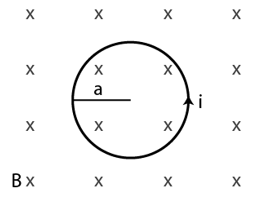Solution:

(a) Magnetic force due to presence of current on a small differential length dl

F = iB dl

B= magnetic field; I = current ; dl =differential length of the wire and

θ = the angle between B and dl

Using Fleming’s left-hand rule is towards the centre for any differential length dl of the wire.

Also, dl and B are perpendicular to each other.

(b) Suppose a part of loop subtends a small angle 2θ at the centre of a circular loop.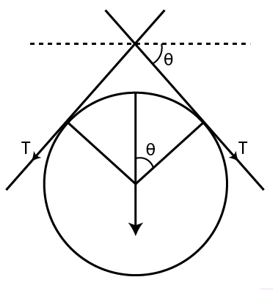From fig.,

2T sinθ = iB dl

and length l of an arc : l = rθ

Here, arc is subtending an angle 2θ, This implies

2T sinθ = iB x 2θ

Since θ is very small so, sinθ = θ.

=> 2Tθ = iB x 2θ

=> T = iB